# Customizing REGIONPROPS With Your Own Measurements

I saw a presentation last month that mentioned a user request to have the ability to customize regionprops. That is, a user wanted to be able to add their own measurement to regionprops.

Today, I'll show you how to do this yourself.

First, here's a brief recap on what regionprops does. The function computes measurements of image regions. Some of these measurements are based purely on a region's shape, while others incorporate pixel values within the regions. Here's an example using the coins.png sample image.

I = imread('coins.png');
imshow(I)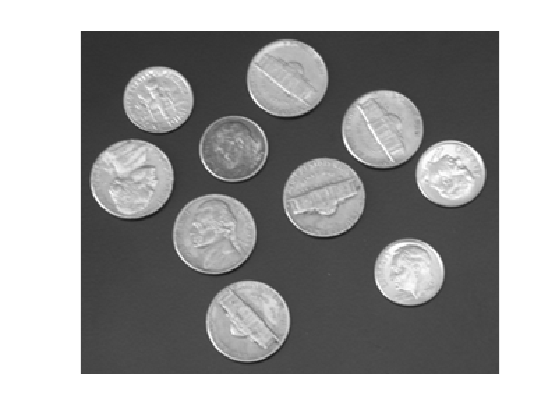Let's convert this image to binary, using adaptive thresholding, filling holes, and removing small "noise" pixels.

bw = imbinarize(I,'adaptive');
bw = imfill(bw,'holes');
bw = bwareafilt(bw,[100 Inf]);
imshow(bw)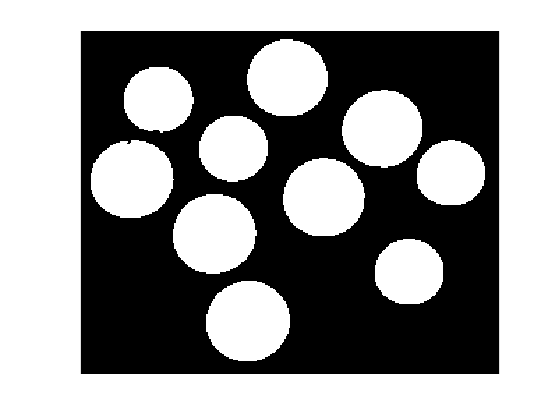You can count the "blobs" (object) yourself; there are 10 of them.

The simplest regionprops call, regionprops(bw) computes the Area, Centroid, and BoundingBox for each object.

s = regionprops(bw)

s =

10×1 struct array with fields:

Area
Centroid
BoundingBox



But I don't think this is the best way to call regionprops anymore. You can now tell regionprops to return the results as a table.

t = regionprops('table',bw)

t =

10×3 table

Area        Centroid        BoundingBox
____    ________________    ____________

2635    37.133    106.85    [1x4 double]
1846    56.131    49.693    [1x4 double]
2672    96.199    146.05    [1x4 double]
1839    109.97    84.848    [1x4 double]
2744    120.37    208.73    [1x4 double]
2520    148.57    34.404    [1x4 double]
2589    174.83    120.01    [1x4 double]
2518    216.81    70.649    [1x4 double]
1857    236.03    173.36    [1x4 double]
1829    265.96    102.64    [1x4 double]



The table form is a lot more convenient for many tasks. For today's topic, one especially nice thing thing about tables is how easy it is to add your own variables to the table.

To illustrate, let's add a measurement that I've seen called Roundness. One definition for roundness is:

$R = \frac{4A}{\pi L^2}$

where $A$ is the object area and $L$ is the major axis length of the best-fit ellipse for the object. Here's how to compute roundness and add it directly to the measurements returned by regionprops.

First, note that both Area and MajorAxisLength are supported by regionprops, so let's start with those.

t = regionprops('table',bw,'Area','MajorAxisLength')

t =

10×2 table

Area    MajorAxisLength
____    _______________

2635     60.08
1846    50.178
2672    59.792
1839    49.674
2744    60.374
2520     58.08
2589    58.676
2518    58.162
1857     49.77
1829    49.564



You access table variables using dot notation, like t.Area. Similarly, you can create a new table variable using dot notation and assignment, like t.MyVariable = .... So adding Roundness to the table returned by regionprops is this simple.

t.Roundness = 4 * t.Area ./ (pi * t.MajorAxisLength.^2)

t =

10×3 table

Area    MajorAxisLength    Roundness
____    _______________    _________

2635     60.08             0.92945
1846    50.178             0.93352
2672    59.792              0.9516
1839    49.674             0.94893
2744    60.374              0.9585
2520     58.08             0.95118
2589    58.676             0.95745
2518    58.162             0.94772
1857     49.77             0.95453
1829    49.564             0.94798



Let's try this computation with an image containing objects that are not quite as round.

I2 = imread('rice.png');
imshow(I2)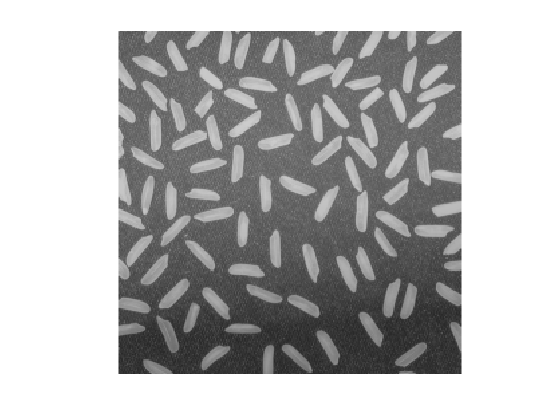bw2 = imbinarize(I2,'adaptive');
bw2 = imfill(bw2,'holes');
bw2 = bwareafilt(bw2,[100 Inf]);
imshow(bw2)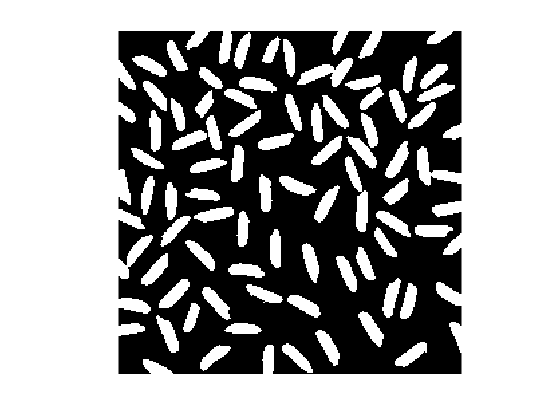t2 = regionprops('table',bw2,'Area','MajorAxisLength');
t2.Roundness = 4 * t2.Area ./ (pi * t2.MajorAxisLength.^2);

ans =

8×3 table

Area    MajorAxisLength    Roundness
____    _______________    _________

138     23.594             0.31562
120     18.152              0.4637
169     28.123             0.27207
157     23.793              0.3531
284     43.757             0.18885
200     26.259             0.36929
141     21.647             0.38311
177     29.087             0.26636



I'm a big fan of the (relatively) new histogram function in MATLAB, so let's use it to compare our roundness numbers. I will follow the advice given in the histogram reference page for normalizing multiple histograms so that they can be more easily compared. I'll set the y-axis limits to [0 1], which is appropriate for probability normalization, and I'll set the x-axis limits to [0 1], which is the range for Roundness.

h1 = histogram(t.Roundness);
hold on
h2 = histogram(t2.Roundness);
hold off
h1.Normalization = 'probability';
h2.Normalization = 'probability';
h1.BinWidth = 0.02;
h2.BinWidth = 0.02;

xlim([0 1]);
ylim([0 1]);

title('Histogram of roundness (probability normalization)')
legend('coins','rice')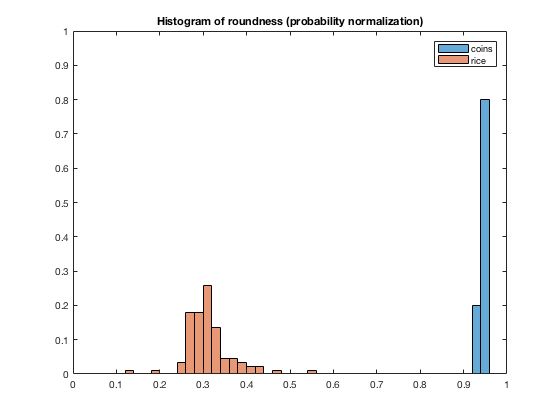There you have it. You can add your own object measurements to the output of regionprops. It's especially easy if you tell regionprops to return a table.

I'll leave you with this question, dear reader: Are there measurements you would like us to add to regionprops? I am aware of an enhancement request for the Feret diameter. What else would you like to see?

Published with MATLAB® R2017a

|

### 댓글

댓글을 남기려면 링크 를 클릭하여 MathWorks 계정에 로그인하거나 계정을 새로 만드십시오.